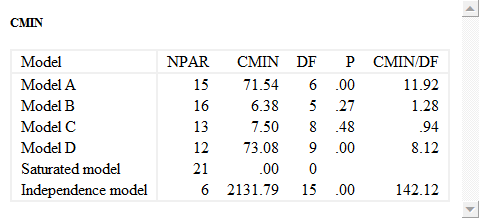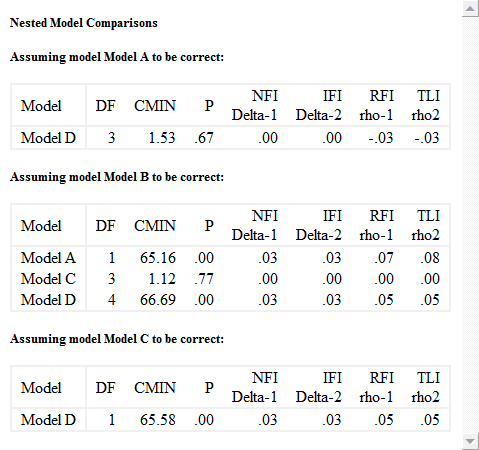﻿ Model comparisons

# Model comparisons

You can fit multiple models in a single analysis. This allows Amos to summarize the results for all models in a single table. The following table is from the output of Example 6 in the user's guide. The table shows the chi square fit statistic (labeled CMIN) for four models called Model A, Model B, Model C and Model D.When Amos can detect that two models are "nested" so that one can be obtained by constraining the other, it performs a chi-square test of the more constrained model under the assumption that the less constrained model is correct. The following tables show the result of testing five separate hypotheses, namely ...

• ... that Model D is correct assuming that Model A is correct.
• ... that Model A is correct assuming that Model B is correct.
• ... that Model C is correct assuming that Model B is correct.
• ... that Model D is correct assuming that Model B is correct.
• ... that Model D is correct assuming that Model C is correct.